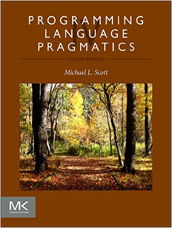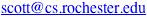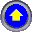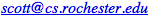## PLP 4e Errata

This page contains a list of all known errors in the text.  To report additional bugs, send mail to### Main book

• Page 54, first line of displayed regular expression at bottom of page: replace “`*` non-`/`” with “non-`*` `/` | `*`+ non-(`*`, `/`)”.
• Page 56, 3rd line of Section 2.2.1: replace “covert” with “convert”.
• Page 84, 8th line up from the bottom: replace “for nonterminals” with “for terminals”.
• Page 99, Figure 2.29, line 8: replace “array [symbol, state]” with “array [state, symbol]”.
• Page 100, Figure 2.30, 25th line of derivation: replace “stmt_list → stmt” with “stmt_list → stmt_list stmt”.
• Page 171, Exercise 3.13, third-to-last line: replace “What does this program print?  What would it print if ...” with “What is the result of evaluating `(A)`?  What would the result be if ...”.
• Page 180, second-to-last line, replace “contract” with “contrast”.
• Page 400, Example 8.57. Replace with
In OCaml, equivalent operations can be found in the `List` library:

 `open List;;` `'a' :: ['b'];;` ⟹ `['a'; 'b']` `hd ['a'; 'b'];;` ⟹ `'a'` `hd [];;` ⟹ run-time exception `tl ['a'; 'b'; 'c'];;` ⟹ `['b'; 'c']` `tl ['a'];;` ⟹ `[]` `tl [];;` ⟹ run-time exception `['a'; 'b'] @ ['c'; 'd']` ⟹ `['a'; 'b'; 'c'; 'd']`

Run-time exceptions may be caught by the program if desired, using techniques
we will cover in Section 9.4.  OCaml programmers are encouraged, however, to
avoid `hd` and `tl` in favor of the `match` construct (Section 11.4.4), which makes it
easier to ensure, at compile time, that run-time exceptions will never occur.

• Page 499, last paragraph of Example 10.28: replace “in languages that use a uniform value model for class-type variables” with “in languages that use a uniform reference model for class-type variables”.
• Page 579, signature for `fold` in Example 11.74: replace
```val fold : ('a * 'b -> 'b) * 'b * 'a list -> 'b = <fun>```” with
```val fold : ('a * 'b -> 'a) * 'a * 'b list -> 'a = <fun>```”.
• Page 691, Exercise 13.9(a): replace “it is not sufficient” with “it suffices”.
• Page 691, Exercise 13.9(b): replace the entire question with “In that same figure, without `volatile` labels, explain why it suffices to enclose each thread’s code in a `synchronized` block for some common shared object O, but it does not suffice to enclose only the pairs of reads in C and D.
• Page 715, Figure 14.3, line 10: replace “`i == 0`” with “`i == 1`”.
• Page 715, one line before Example 14.21: replace “`NR`” with “`NF`”.
• Page 786, Figure 15.6:
1. replace the current productions for read, write, and writeln withcall : stmt1 → id expr stmt2
• expr.next_free_reg := stmt2.next_free_reg := stmt1.next_free_reg
• stmt1.code := expr.code + [“a1 :=” expr.reg] + [“call” id.stp→symbol] + stmt2.code
call : expr1 → id expr2
• expr2.next_free_reg := expr1.next_free_reg
• expr1.reg := reg_names[expr1.next_free_reg mod k]
expr1.code := expr2.code + [“a1 :=” expr2.reg] + [“call” id.stp→symbol] + [expr1.reg “:= rv”]
null : expr → ε
• expr.reg := “r1”       −− harmless0
expr.code := null2. in the productions for assign and id, replace “stp→name” with “stp→symbol”.
3. in the productions for <>, >, and , erase the right-triangle glyph.
• Page 787, Figure 15.6 (continued): replace the first four lines withmacro handle_op(ref result, L_operand, R_operand : syntax_tree_node; op : string)
• L_operand.next_free_reg := result.next_free_reg0
R_operand.next_free_reg := result.next_free_reg + 10
• result.reg := L_operand.reg
• Page 789, Figure 15.7:
1. replace lines 8–14 (the ones right after “main:”) with
a1 := r1         −− harmless
call getint      −− “getint” and “putint” are library subroutines
−− to be found by the linker
r1 := rv
i := r1
a1 := r1         −− harmless
call getint
r1 := rv
j := r1
2. replace the last 7 lines with
r1 := i
a1 := r1
call putint
NB: Errata on pages 786, 787, and 789 “go together.”  Corrected images of these three pages are available HERE.
• Page 790: delete “Check Your Understanding” question number 7.  (The answer is no longer covered in this edition.)
• Page 811: second full paragraph, line 10: replace “easily if” with “easily than it could if”.

### Companion site

• Page C-346, Figure 17.19:  replace “v19 with “v19 ; replace “v26 with “v26 . r4” r0” r5” r1”
• Page C-346, Figure 17.20,  left column, line 3: replace “∗r5 := 1” with “∗r1 := 1” ;left column, lines 8 & 9: replace “r5 := r4 << 1 with “r5 := r0 << 1 ; r3 := r4” r3 := r0”left column, line 14: replace “r6 := r4 div r2” with “r6 := r0 div r2” ;right column, line 1: replace “r5 := r5 + 4” with “r1 := r1 + 4” ;right column, lines 3 & 4: replace “r4 := r6 x r3 with “r0 := r6 x r3 ; ∗r5 := r6” ∗r1 := r6”right column, lines 10 & 11: replace “r6 := r4 div r2 with “r6 := r0 div r2 . ∗(r5+4) := r6” ∗(r1+4) := r6”NB: A corrected image of page C-346 is available HERE.

Thanks to Carl Albing, Wentao Cai, Nicholas Coleman, Chen Ding, Samuel Estep, Alexander Gutierrez, Lucian Ilie, Shuo (Max) Li, Sreepathi Pai, Colin Pronovost, Ben Steele, Yanling Wang, and Zhizhou Zhang for their help in catching these mistakes.Back to the book home page
Last Change: 24 January 2022 /